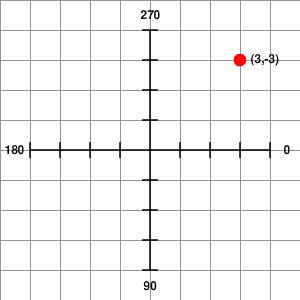# Circular Motion in AS3: Make One Moving Object Orbit AnotherThis post is part of a series called You Do The Math.
Hit the Target With a Deadly Homing Missile
Understanding Affine Transformations With Matrix Mathematics

Having one object orbit another is a movement mechanic that's been used since the early gaming era, and it remains handy to this very day! In this Quick Tip we will explore the mathematical function for orbiting, see how to modify it, and look at practical uses in actual game design.

## Final Result Preview

Here's what we will be working towards:

## Step 1: The Orbiting Equation

To create an orbit we must define the following:

• Origin - The object or position that the orbit centers on.
• Orbiter - The object that orbits the origin.
• Angle - The current angle of the orbiter.
• Speed - The number of pixels our object orbits per frame.
• Radius - The orbiter's distance from the origin.

It is also helpful to note that Flash's coordinate system is like a regular cartesian plane, except that the y-axis is flipped, so the value increases as we move downwards. The top-left corner of the stage has coordinates (0,0)In this image, 0, 90, 180, and 270 refer to angles, measured in degrees.

Another thing that we need to understand is how to convert degrees to radians, which can easily be accomplished using the following formula:

Radians = Degrees * (PI / 180)

Here's the actual orbiting function: it requires two lines, one to position the orbiter on the x-axis and the other to position it on the y-axis:

(In a normal Cartesian plane, sin is used for the x-coord and cos is used for the y-coord, but since our angles are increasing clockwise - due to the reversed y-axis - their places are swapped.)

## Step 2: Writing the Equation in Code

We can now turn the logic into actual code that we can use. First, declare all of the variables:

 1 2 public var orbiter:Orbiter = new Orbiter(); // The MovieClip That Orbits  3 public var origin:Origin = new Origin(); // The MovieClip That Is Orbited  4 public var angle:Number = 0; // The Initial Angle Orbiting Starts From  5 public var speed:Number = 3; // Number Of Pixels Orbited Per Frame  6 public var radius:Number = 75; // Orbiting Distance From Origin 

Next rewrite the equation for use in AS3 and put it into an ENTER_FRAME event handler function:

 1 2 var rad:Number = angle * (Math.PI / 180); // Converting Degrees To Radians  3 orbiter.x = origin.x + radius * Math.cos(rad); // Position The Orbiter Along x-axis  4 orbiter.y = origin.y + radius * Math.sin(rad); // Position The Orbiter Along y-axis 

If you test now, nothing will happen; this is because the variable angle is neither increasing nor decreasing. Therefore, we must increase or decrease the value:

 1 2 angle += speed; // Object will orbit clockwise  3 angle -= speed; // Object will orbit counter-clockwise 

Now what if we want our orbiter to continuously face a direction? Well I have written an equation to do just that!

 1 2 orbiter.rotation = (Math.atan2(orbiter.y-origin.y, orbiter.x-origin.x) * 180 / Math.PI); 

Depending on which way you have drawn your orbiter movieclip you may have to subtract a certain angle (outside the brackets) to get it to face the correct way.

## Step 3: Transforming the Equation

Now this may sound crazy, but some of us might want to have our object orbit in an ellipse. This is easily doable; all we have to do is multiply in a specific place in the equation. Multiplying the cos or sin functions by a postive whole number will cause the circle to stretch. Multiplying it by a decimal between 0 - 1 will cause it to compress. And multiplying it by a negative will cause it to flip along that axis:

 1 2 (2 * Math.cos(rad)); // Stretch the orbit along the x-axis by a factor of 2  3 (0.5 * Math.sin(rad)); // Compress the orbit along the y-axis by a factor of 2 (i.e. halve it)  4 (-3 * Math.cos(rad)); // Flip the orbit along the x-axis and stretch it by a factor of 3 

We can also shift the orbit in any direction we want by adding or subtracting from the equation on either axis:

 1 2 orbiter.x = (origin.x + radius * Math.cos(rad))-50; // Shift the orbit 50 pixels left on the x-axis 

If you want to learn more about cos, sin, and atan2, take a look at Trigonometry for Flash Developers.

## Step 4: Practical Uses for the Equation

Now this is all fine and dandy, but what can it actually be used for? A wide variety of things actually!

If you have ever played Mario Kart you would have gotten the "three shells" powerup; those shells are orbiting using this very technique. Another example is the widely over-used circle pong game, where the paddle orbits along a ring on the outside. A third example is from top down zombie shooter games: a lot of them include a powerup where a grouping of barrels orbits around your player and crushes any zombies if they are hit trying to attack you.

As you can see the use for this technique can be used in anything from industry standard games to casual games.

## Conclusion

Thank you for taking the time to learn this Quick Tip! If you have any questions leave a comment below.